# 最大二叉树 II

## 998. 最大二叉树 II (Medium)

• 如果 `A` 为空，返回 `null`
• 否则，令 `A[i]` 作为 A 的最大元素。创建一个值为 `A[i]` 的根节点 `root`
• `root` 的左子树将被构建为 `Construct([A, A, ..., A[i-1]])`
• `root` 的右子树将被构建为 `Construct([A[i+1], A[i+2], ..., A[A.length - 1]])`
• 返回 `root`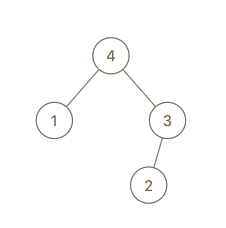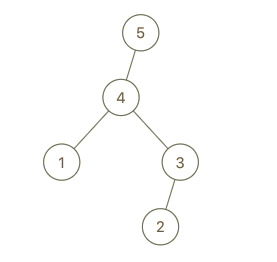```输入：root = [4,1,3,null,null,2], val = 5

```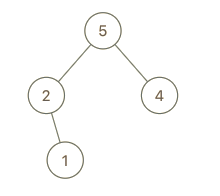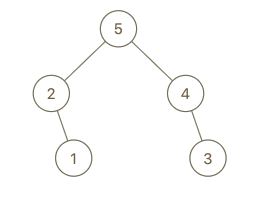```输入：root = [5,2,4,null,1], val = 3

```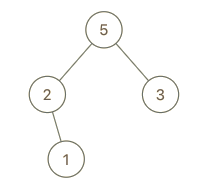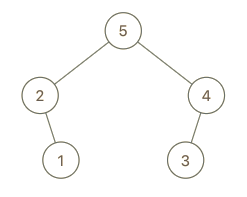```输入：root = [5,2,3,null,1], val = 4

```

1. `1 <= B.length <= 100`

[]

### 相似题目

1. 最大二叉树 (Medium)